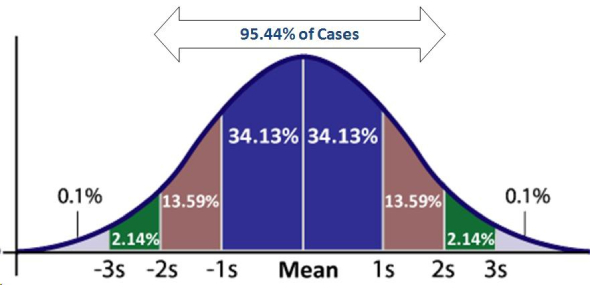# Probability Distribution Test: Quiz!

Approved & Edited by ProProfs Editorial Team
At ProProfs Quizzes, our dedicated in-house team of experts takes pride in their work. With a sharp eye for detail, they meticulously review each quiz. This ensures that every quiz, taken by over 100 million users, meets our standards of accuracy, clarity, and engagement.
| Written by Hdagle
H
Hdagle
Community Contributor
Quizzes Created: 1 | Total Attempts: 1,145
Questions: 9 | Attempts: 1,149Settings• 1.

• 2.

• 3.

• A.

-1.85

• B.

0.15

• C.

0.6

• D.

1.85

B. 0.15
• 4.

### Create a probability distribution table showing the assignment of final grades and grade-point scores. An instructor always assigns final grades such that 20% are A, 40% are B, 30% are C, and 10% are D. The grade-point scores are 4 for A, 3 for B, 2 for C, and 1 for D.

Grade A B C DGrade Point Score 4 3 2 1P(x) .20 .40 .30 .10 A B C D.2 .4 .3 .14 3 2 1
Explanation
The probability distribution table shows the assignment of final grades and grade-point scores. The table displays the percentages of each grade (A, B, C, and D) and their corresponding grade-point scores (4, 3, 2, and 1). The values in the table represent the probabilities of each grade occurring. For example, there is a 20% probability of getting an A, a 40% probability of getting a B, a 30% probability of getting a C, and a 10% probability of getting a D. The table also shows the grade-point scores associated with each grade.

Rate this question:

• 5.

### In a family with two children, find the mean of the number of children who will be girls. Number of girls, X 0 1 2 Probability, P(X) ¼ ½ ¼

• A.

0

• B.

1/4

• C.

1/2

• D.

1

D. 1
Explanation
The mean of the number of children who will be girls can be calculated by multiplying each number of girls by its corresponding probability and summing them up. In this case, we have 0 girls with a probability of 1/4, 1 girl with a probability of 1/2, and 2 girls with a probability of 1/4. Therefore, the mean is (0 * 1/4) + (1 * 1/2) + (2 * 1/4) = 1.

Rate this question:

• 6.

### Dropping College Courses Use the following table to answer the question. Would you consider the information in the table to be a probability distribution? Reason for Dropping a College Course Frequency Percentage Too difficult 45   Illness 40   Change in work schedule 20   Change in major 14   Family-related problems 9   Money 7   Miscellaneous 6   No meaningful reason 3

• A.

Yes, you can find the probabilties.

• B.

Yes, you only need frequency

• C.

No, the probabilities are not listed.

• D.

No, there is not enough information to find the probabilities.

C. No, the probabilities are not listed.
Explanation
The table provides the frequency of each reason for dropping a college course, but it does not provide the probabilities. In order to have a probability distribution, the probabilities of each event must be listed. In this case, only the frequencies are given, so the probabilities cannot be determined.

Rate this question:

• 7.

### The mean for a probability distribution is the same as the expected value of a discrete random variable of a probability distribution.

• A.

True

• B.

False

A. True
Explanation
The statement is true because the mean of a probability distribution represents the average value of the random variable, and the expected value of a discrete random variable is also a measure of the average value. Therefore, they are equivalent and represent the same concept in probability theory.

Rate this question:

• 8.

### Tossing a Die Use the table below to answer the question. Find the standard deviation of the number of spots that will appear when a die is tossed. In the toss of a die, the probability distribution for the number of spots that appear is shown below. Outcome, X 1 2 3 4 5 6 Probability, P(X) 0.167 0.167 0.167 0.167 0.167 0.167

• A.

0.167

• B.

1.667

• C.

1.70

• D.

2.9

C. 1.70
Explanation
The standard deviation is a measure of the variability or spread of a probability distribution. In this case, the probability distribution for the number of spots that appear when a die is tossed is uniformly distributed, meaning that each outcome has an equal probability of occurring. The standard deviation for a uniform distribution can be calculated using the formula sqrt((n^2 - 1)/12), where n is the number of possible outcomes. In this case, n = 6, so the standard deviation is sqrt((6^2 - 1)/12) = sqrt(35/12) ≈ 1.70. Therefore, the correct answer is 1.70.

Rate this question:

• 9.

### Expected Value A person selects a card from the deck. If it is a red card, she wins \$1. If it is a black card between or including 2 and 10, she wins \$5. If it is a black card, she wins \$10; and if it is a black ace, she wins \$100. Find the expectation of the game. How much should a person bet if the game is to be fair?Back to top